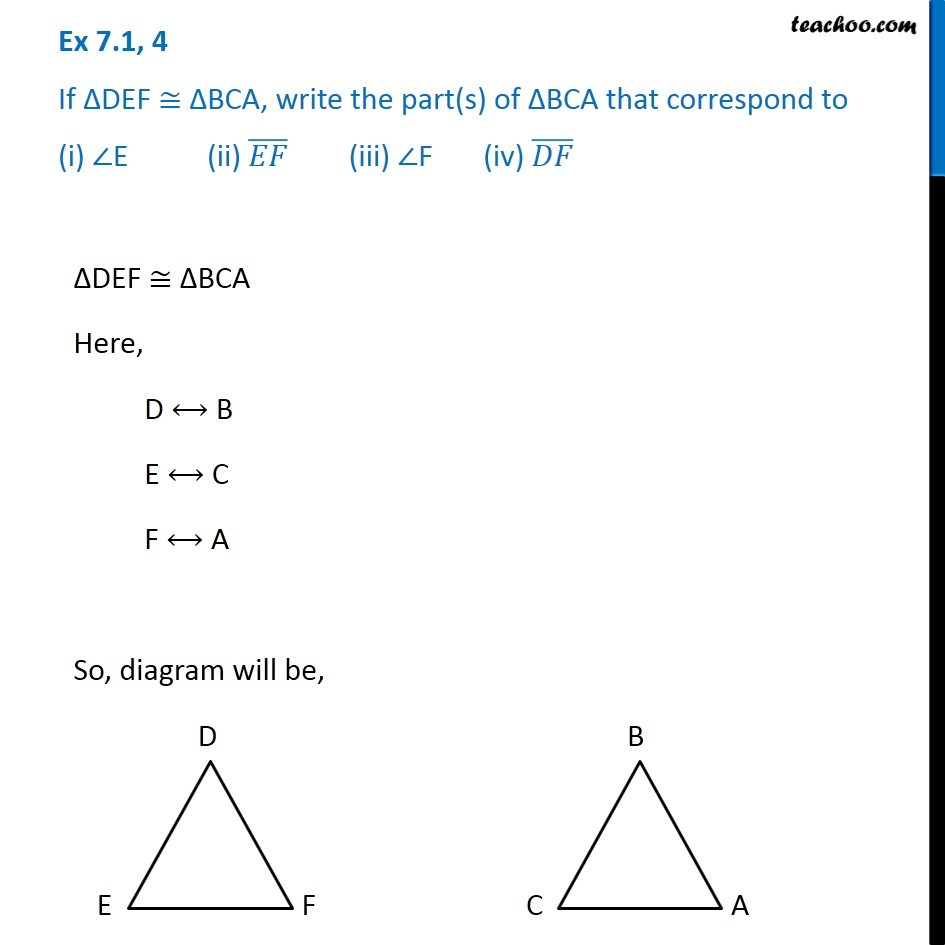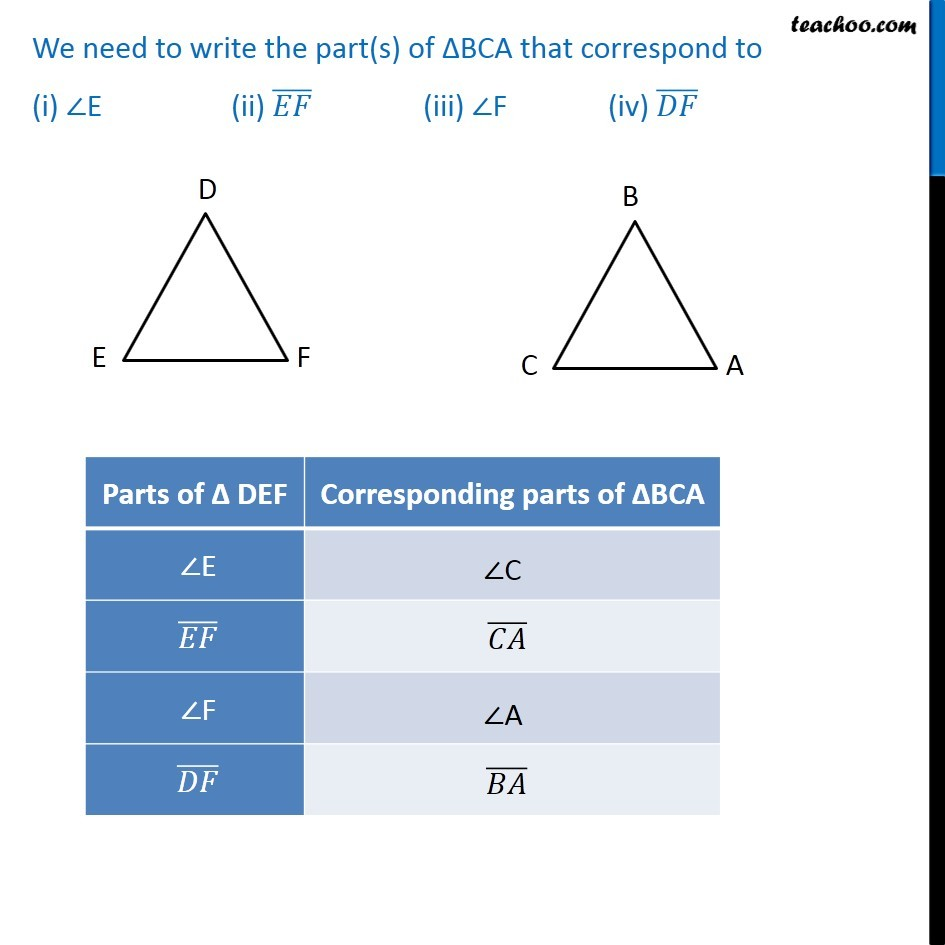1. Chapter 7 Class 7 Congruence of Triangles
2. Concept wise
3. Congruency of triangles

Transcript

Ex 7.1, 4 If ∆DEF ≅ ∆BCA, write the part(s) of ∆BCA that correspond to (i) ∠E (ii) (𝐸𝐹) ̅ (iii) ∠F (iv) (𝐷𝐹) ̅ ∆DEF ≅ ∆BCA Here, D ⟷ B E ⟷ C F ⟷ A So, diagram will be, Corresponding parts of > angle E is angle C > EF is CA > angle F is angle A > DF is BA